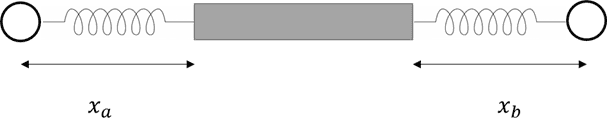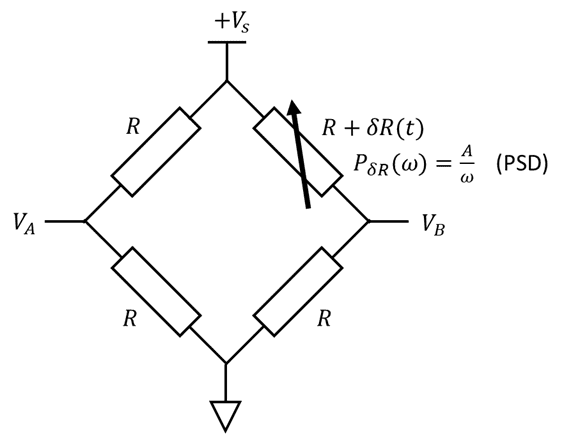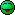# Experimental Physics Challenge, June 2021

• Challenge
Science Advisor
Gold Member
Trying this out for fun, and seeing if people find this stimulating or not. Feedback appreciated! There's only 3 problems, but I hope you'll get a kick out of them. Have fun!

1. Springey Thingies:Two damped, unforced springs are weakly coupled and obey the following equations of motion: $$\ddot{x}_a +\gamma \dot{x}_a + \omega_{0,a}^2 x_a + \beta^2 (x_b - x_a) = 0$$ $$\ddot{x}_b +\gamma \dot{x}_b + \omega_{0,b}^2 x_b - \beta^2 (x_b - x_a) = 0$$ You wish to measure the difference between the two springs' natural (undamped) resonant frequencies: ##\Delta = \omega_{0,b} - \omega_{0,a}##. Your measurement will be complicated by the coupling coefficient β and the damping coefficient γ. Design a simple procedure for measuring ##\Delta##.

Assume for simplicity that ##\omega_0 = \frac{1}{2}\left(\omega_{0,b} + \omega_{0,a}\right) = 1\mathrm{kHz}## and ##\gamma = 125\mathrm{s^{-1}}## are known exactly. You are also given that Δ is of order ##2\pi\times 1\mathrm{mHz}## and β is of order ##2\pi\times100\mathrm{mHz}##. The uncertainty in either spring's measured position is determined by the spring's initial conditions by $$\sigma_x = \left(2.6\times10^{-7}\right)\sqrt{x(0)^2 + \frac{\dot{x}(0)^2}{\omega_0^2 - \gamma^2 / 4}}$$
Your answer should include a set of times at which to measure the spring positions ##x_a## and ##x_b##, a formula for ##\Delta## in terms of these measurements, and a standard deviation on the value of ##\Delta##. Optimal answers should have uncertainty ##\sigma_\Delta \approx 2\pi \times 10\mathrm{\mu Hz}## with as little as two position measurements. Numerical and analytical methods are accepted so long as the results are valid!

2. "Honey, I shrunk the error bars!"

You and your coworker Bob are studying a chemical reaction ##A + B \leftrightarrow C##. For this study, you vary the temperature of the mixture and record the concentration of species C: $$x = \frac{N_C}{N_A + N_B + N_C}$$ where ##N_A##, ##N_B##, and ##N_C## refer to the total number of each species A, B, and C respectively. For each temperature setting, you record a number (M) of measurements of ##N_A##, ##N_B##, ##N_C## (M measurements of each). Furthermore, you know that ##N_A##, ##N_B##, and ##N_C## are all Poisson distributed. You then calculate an average ##\mathrm{E}[x]## and standard error ##\sigma_{\mathrm{E}[x]}## for each set of measurements. Up to this point, everything makes sense.

Your coworker Bob comes up with a wacky idea. Bob re-defines the concentration (now called ##x'##) within a set of M measurements as follows: $$x'_i = \frac{N_{C,i}}{\mathrm{E}[N_A] + \mathrm{E}[N_B] + N_{C,i}} \; \; \mathrm{for} \; i=1,2,...,M$$ Bob argues that taking expectation values over ##N_A## and ##N_B## in the denominator eliminates extraneous noise. What's more, Bob has a mathematical proof that shows that ##\mathrm{Var}[x']\leq\mathrm{Var}[x]##. You make a bet with Bob: you collect 100 data sets, each consisting of M measurements, and compare the estimated standard error on the mean ##\sqrt{\frac{1}{M}\mathrm{Var}[x']}## (aka the "error bars" on the mean of each set of M measurements) with the observed standard deviation on the means of each of the 100 sets of measurements ##\sigma_{\mathrm{E}[x']}##. The data shows that ##\sigma_{\mathrm{E}[x']} > \sqrt{\frac{1}{M}\mathrm{Var}[x']}##, and more specifically that $${\sigma_{\mathrm{E}[x']}}=\sigma_{\mathrm{E}[x]}$$ This last result can be interpreted to mean there is no free lunch for Bob. Reproduce Bob's proof that ##\mathrm{Var}[x']\leq\mathrm{Var}[x]## and prove the "no free lunch" result ##\sigma_{\mathrm{E}[x']}=\sigma_{\mathrm{E}[x]}##.

3. Pink, pink, you stink!

Consider the following bridge circuit, where the variable resistor sees "pink" noise (aka 1/f noise):All 4 resistors have identical resistance on average, but the top right resistor fluctuates with a pink spectrum: $$P_{\delta R}(\omega) = \frac{A}{\omega}$$ where ##P_x(\omega)## is the power spectral density (PSD) of the function ##x(t)##. Each resistor also puts out thermal noise (Johnson-Nyquist noise). Find an expression in terms of the measured voltages ##V_A##, ##V_B##, and ##V_S## that is proportional to the fluctuating resistance ##\delta R## but is independent of thermal noise.

Some sample data is attached (filename is “pinkdatafinalfinal.csv”), where each voltage (##V_A##,##V_B##, and ##V_S##) is reported versus time in a CSV format. Extract the constant A as defined above and state your uncertainty on A, given ##R = 1\mathrm{\Omega}##. My solution has uncertainty on the order of ##1 \times 10^{-8} \mathrm{\mathrm{\Omega^2}}##. There are many methods for tackling this problem and some give higher precision than others.

#### Attachments

• pinkdatafinalfinal.csv
513.7 KB · Views: 14
••berkeman, yucheng, JD_PM and 7 others

## Answers and Replies

Dale
Mentor
2020 Award
Thanks for hosting it this month @Twigg

Anyone who would like to host a future month, just let me know by private message

Bumping this challenge! Too tough for everyone?Science Advisor
Gold MemberI probably went a little overboard with the length and complexity of each problemHere are some hints if y'all are still interested!

The most efficient way to do this problem is to write a quick and dirty numerical program to directly integrate these equations of motion and play around with it. I did this with scipy's odeint function (attached below for all to use). Play around by plotting some different linear combinations of ##x_a## and ##x_b## for different initial conditions, and you'll start to see something magical happening! At that point, you'll know what to look for in the analysis.
Springumathings:
from scipy.integrate import odeint
import numpy as np
import matplotlib.pyplot as plt

# Define physical constants
w0 = 2*np.pi*1000 #average resonant frequency (omega_0) in radians per second
g = 125 #damping rate (gamma) in inverse seconds
B = 2*np.pi*0.5 #coupling rate (beta) in radians per second
D = 2*2*np.pi*4E-3 #difference in resonant frequencyes (Delta) in radians per second

w0a = w0-0.5*D #resonant frequency of the first spring
w0b = w0+0.5*D #resonant frequency of the second spring

def odes(x,t): #return first derivatives in time of the positions and velocities of each spring
dxdt0 = -(g*x + (w0a**2 - B**2)*x + (B**2)*x) #calculate acceleration of the first spring
dxdt1 = -(g*x + (w0b**2 - B**2)*x + (B**2)*x) #calculate acceleration of the second spring
dxdt2 = x #calculate velocity of the first spring
dxdt3 = x #calculate velocity of the second spring
return [dxdt0,dxdt1,dxdt2,dxdt3]

x0 = [0,0,1,0] #initial condition of the two springs in the following format:
#x0 is the velocity of the first spring in meters per second
#x0 is the velocity of the second spring in meters per second
#x0 is the position of the first spring in meters
#x0 is the position of the third spring in meters

# declare a time vector
num_samples = 200 #resolution of the time vector
t = np.linspace(0,2/g,2*num_samples) #create the time vector in seconds, out to 2 decay times as an example

#Integrate!
x = odeint(odes,x0,t)

#Make some plots
fig, axes = plt.subplots(1,2)

ax1 = axes
ax2 = axes

ax1.plot(t,x[:,2])
ax2.plot(t,x[:,3])
ax1.set_xlabel('Time (s)')
ax2.set_xlabel('Time (s)')
ax1.set_ylabel('x_a (m)')
ax2.set_ylabel('x_b (m)')
The starting point here is to express each numeric variable as ##N = \mathrm{E}[N] + \delta N## where ##\delta N## represents fluctuations around the mean. Then you'll want to series expand x and/or x' to first order (since the quantities are Poisson distributed, you know that ##\frac{\sigma_N}{N} = \frac{1}{\sqrt{N}}##, and since this is chemistry-based you can expect ##N## to be ginormous, so there's no harm in truncating to first order).
Any circuit analysis of your favorite variety (kirchoff, nodal, mesh, whatever) will reveal that even the thermal noise on the voltages ##V_A##, ##V_B##, and ##V_S## are correlated. A little strategy and a quick first order series expansion will show that there is a simple function of these voltages that is independent of noise and scales like ##C\delta R + O(\delta R^2)## for some constant C.

•Greg Bernhardt, JD_PM and Dale
Science Advisor
Gold Member
Been a week, so I thought I might do a second round of hints

Try the initial condition ##x_a(0) = x_b(0) = 1## and plot the difference in positions ##x_b(t) - x_a(t)##. Notice any patterns? Try changing the value of ##\Delta## in the simulation and seeing how that plot changes. Since you know the damping rate ##\gamma## exactly, you can even look at the quantity ##e^{+\gamma t} (x_b(t) - x_a(t))## to see the effect minus damping without introducing any error.
Notice that when calculating the variance ##\mathrm{Var}[x']## within a set of M measurements, the mean values ##\mathrm{E}[N_A]## and ##\mathrm{E}[N_B]## are constants, but when comparing multiple sets of M measurements these expectation values are random variables with uncertainty given by the standard deviations ##\sigma_{\mathrm{E}[N_A]}## and ##\sigma_{\mathrm{E}[N_B]}## respectively.
Try looking at the difference ##V_A - V_B##, and taking a first order series expansion in ##\delta R##. This quantity still has thermal noise multiplied in, but there's a way to get rid of it using ##V_S##. To do the spectral density estimate, a Welch periodogram is your best bet. From there, it's just a matter of regression using whatever numerical method you like.